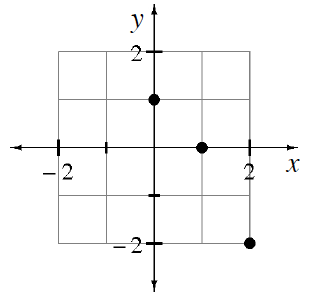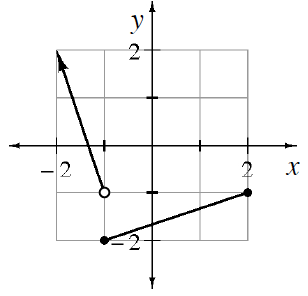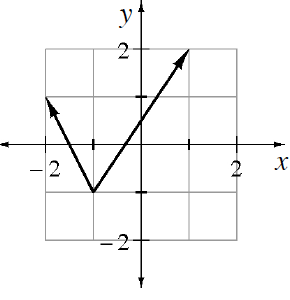### Home > A2C > Chapter 3 > Lesson 3.1.5 > Problem3-71

3-71.

Find the domain and range for each of the relations graphed below.

1.Look in your notes, the glossary, or the index for definitions and examples of domain and range.

Domain: $(0, 1, 2)$
Range: $(−2, 0, 1)$

1.See part (a).

Domain: $-1 ≤ x ≤ 1$
Range: $-1 ≤ y ≤ 2$

1.Domain: $x ≤ 2$
Range: $y ≥ −2$

1.Domain: all real numbers
Range: $y ≥ −1$